Homework Help Question & Answers

# Question 3 Consider the ordinary differential equation (ODE) 2xy" + (1 + x)y' + 3y =...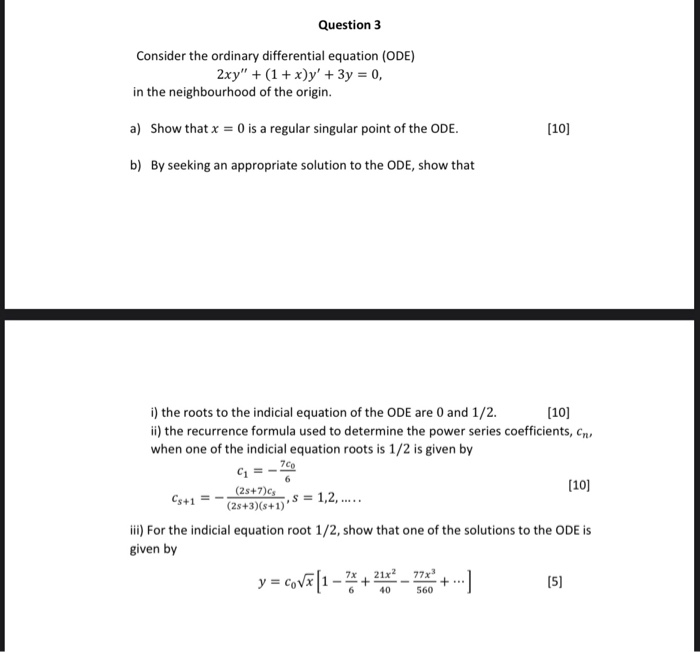Question 3 Consider the ordinary differential equation (ODE) 2xy" + (1 + x)y' + 3y = 0, in the neighbourhood of the origin. a) Show that x = 0 is a regular singular point of the ODE. (10) b) By seeking an appropriate solution to the ODE, show that G=- (10) i) the roots to the indicial equation of the ODE are 0 and 1/2.  ii) the recurrence formula used to determine the power series coefficients, ens when one of the indicial equation roots is 1/2 is given by 7.00 (28+7) Cs+1 s = 1,2,..... (28+3)(8+1) iii) For the indicial equation root 1/2, show that one of the solutions to the ODE IS given by y = cova[1 -+ 21x2_77x (5) 40 560

#### Homework Answers

Answer #1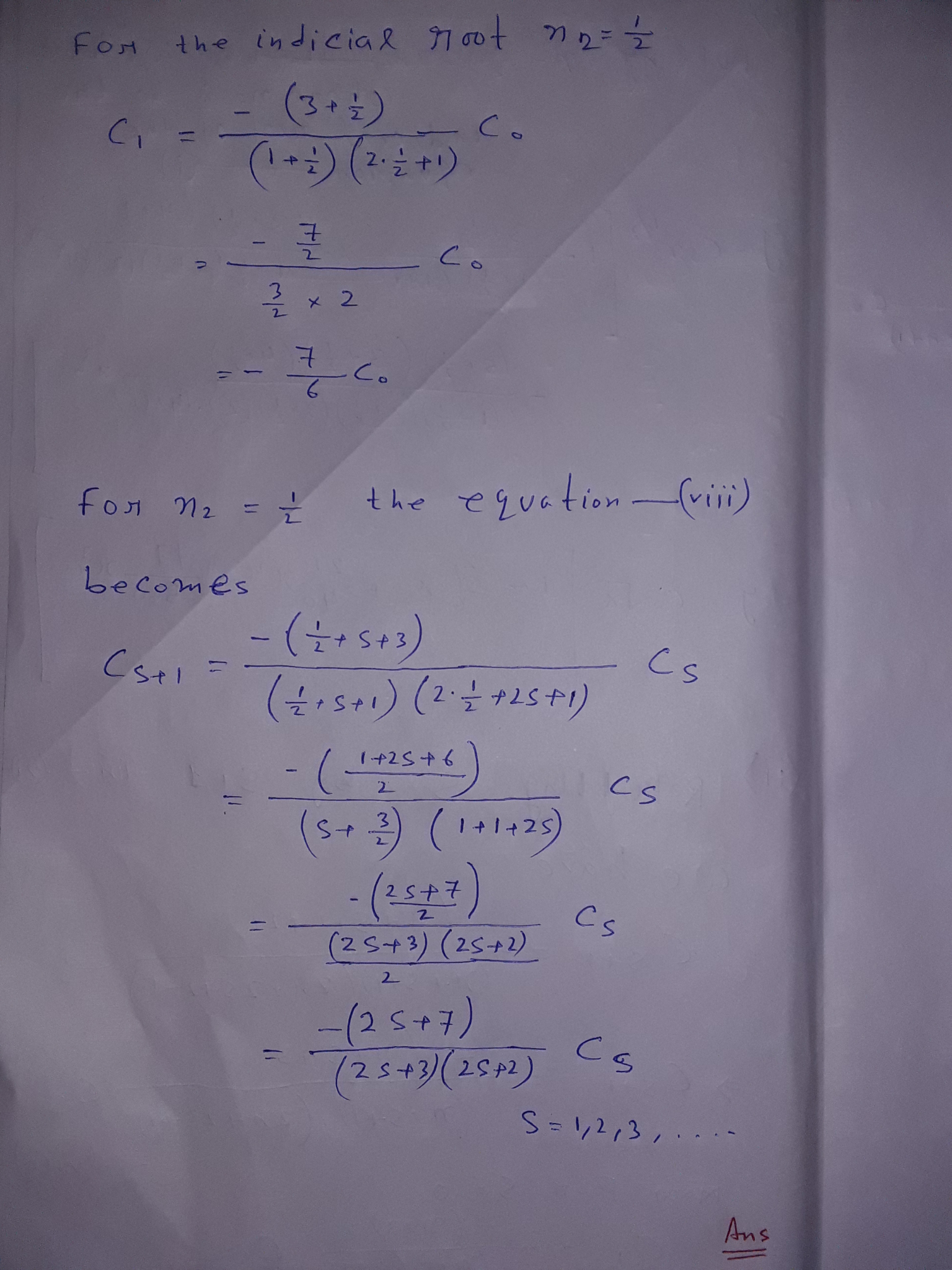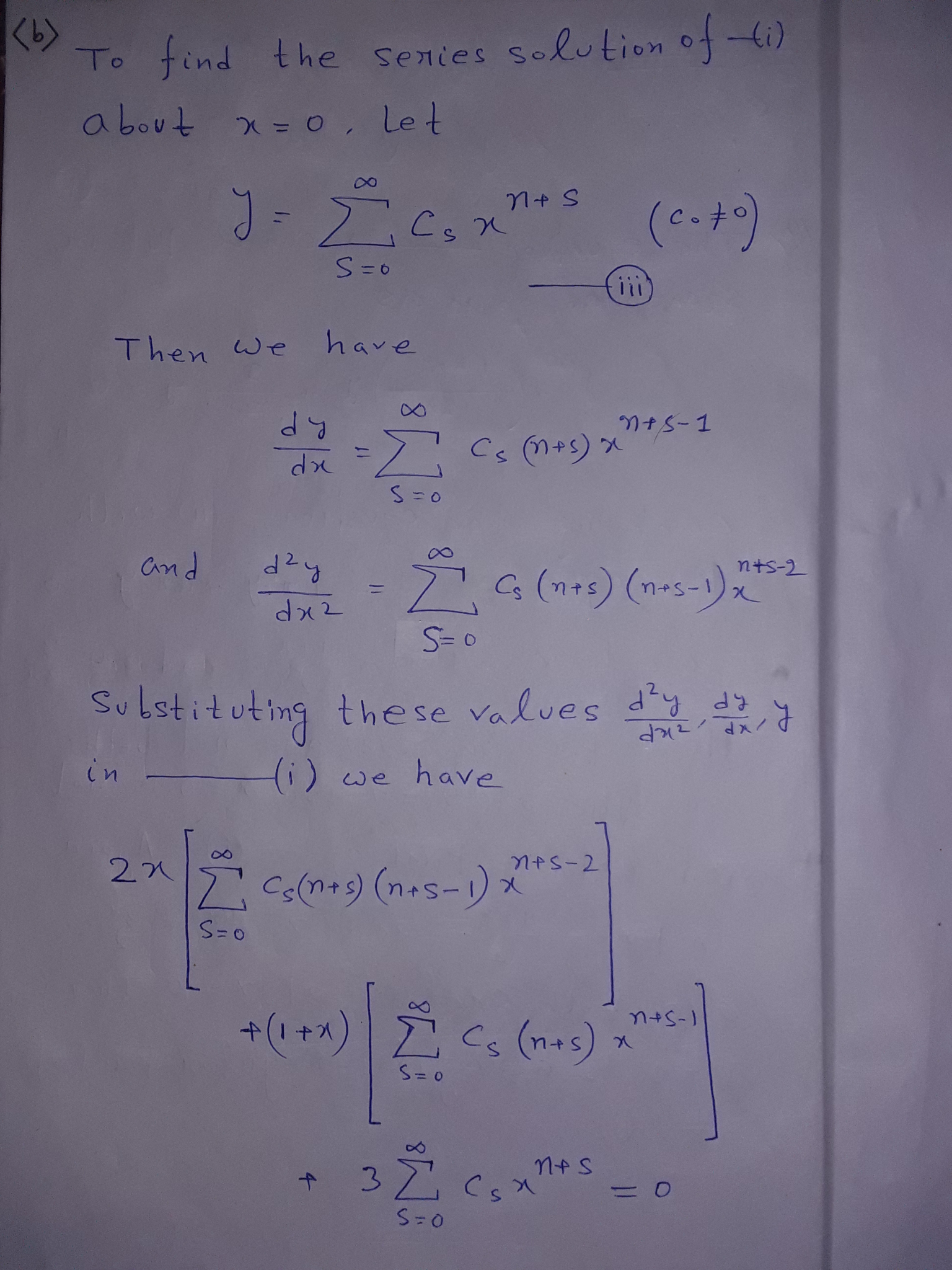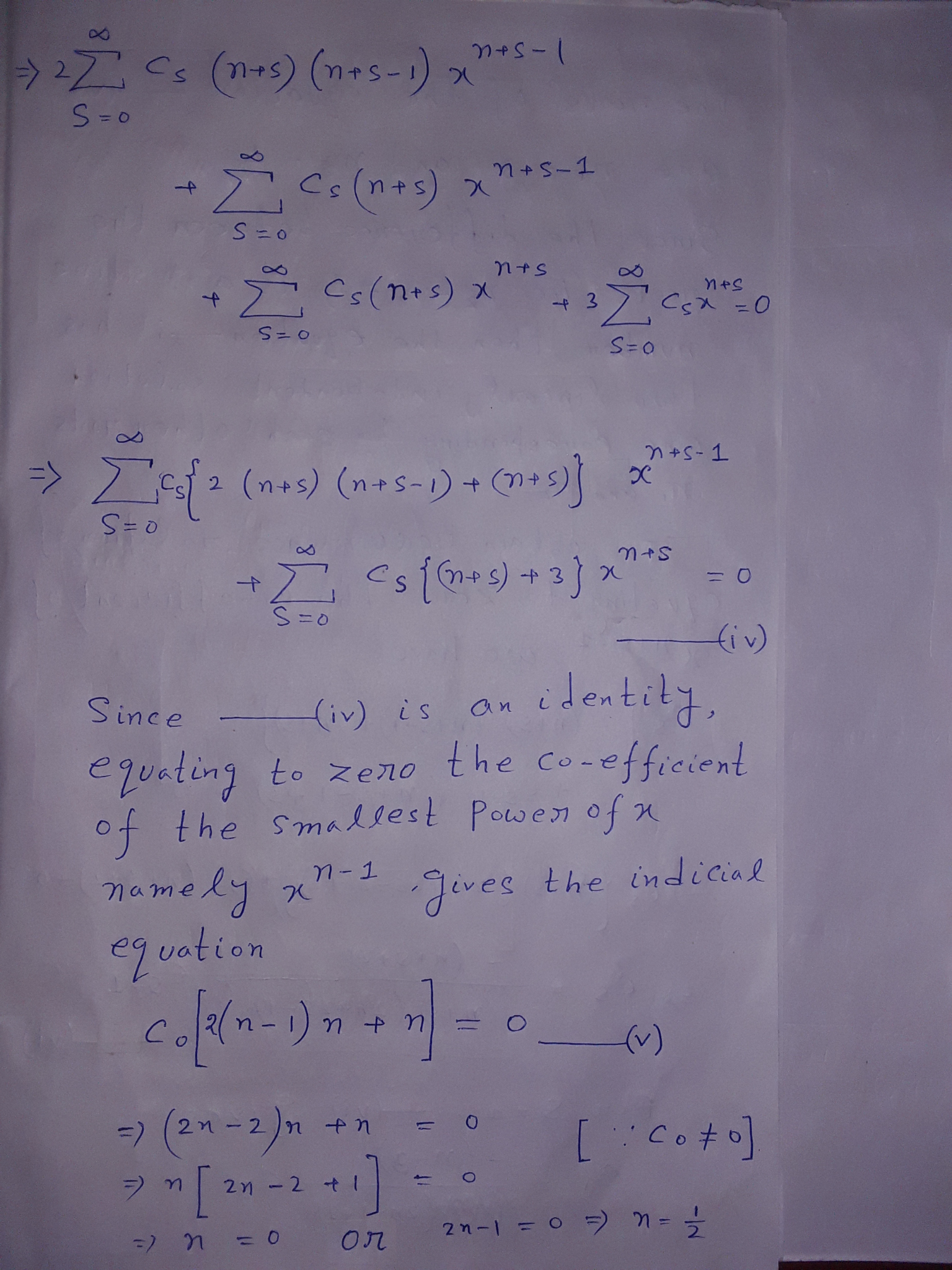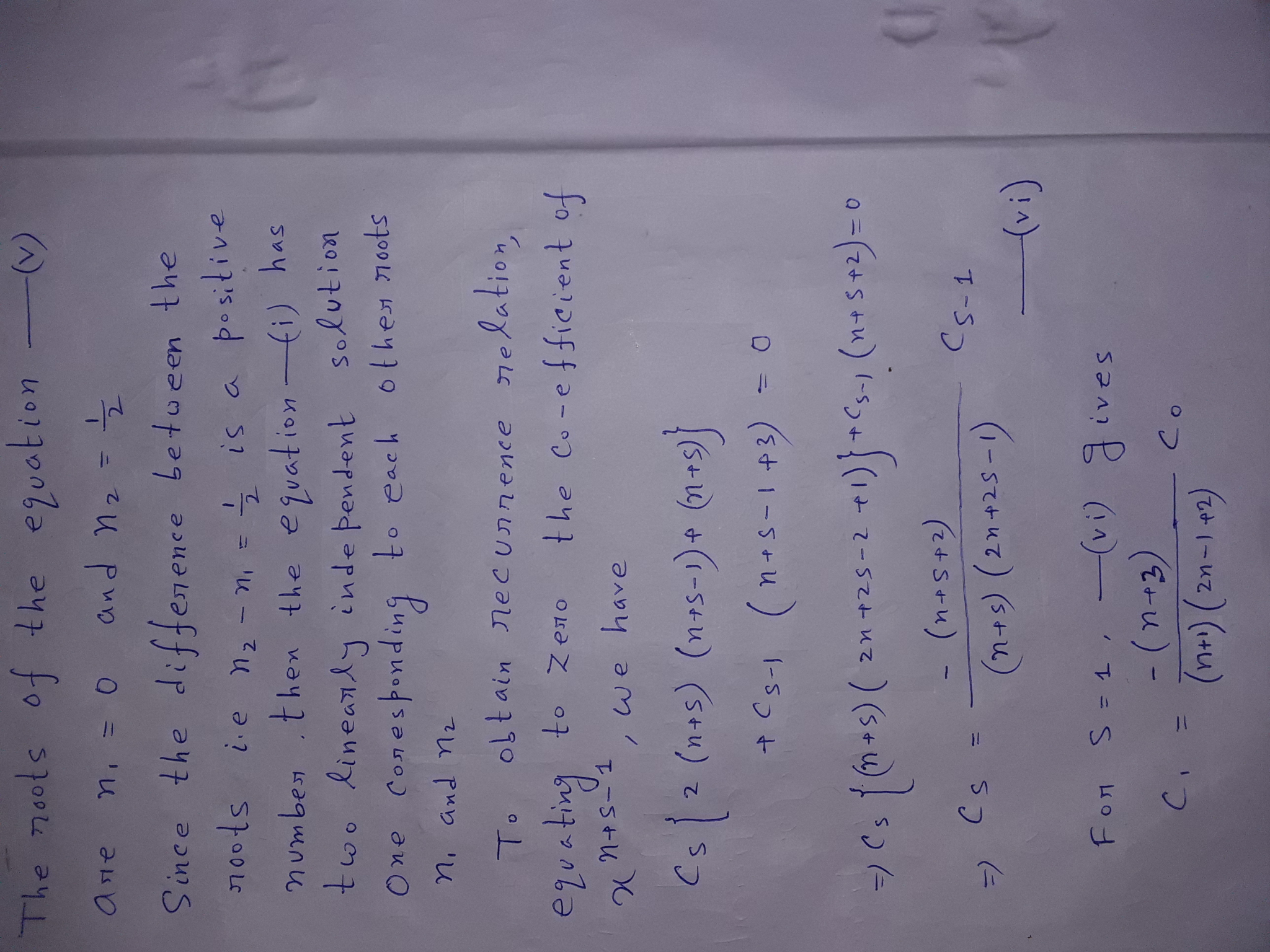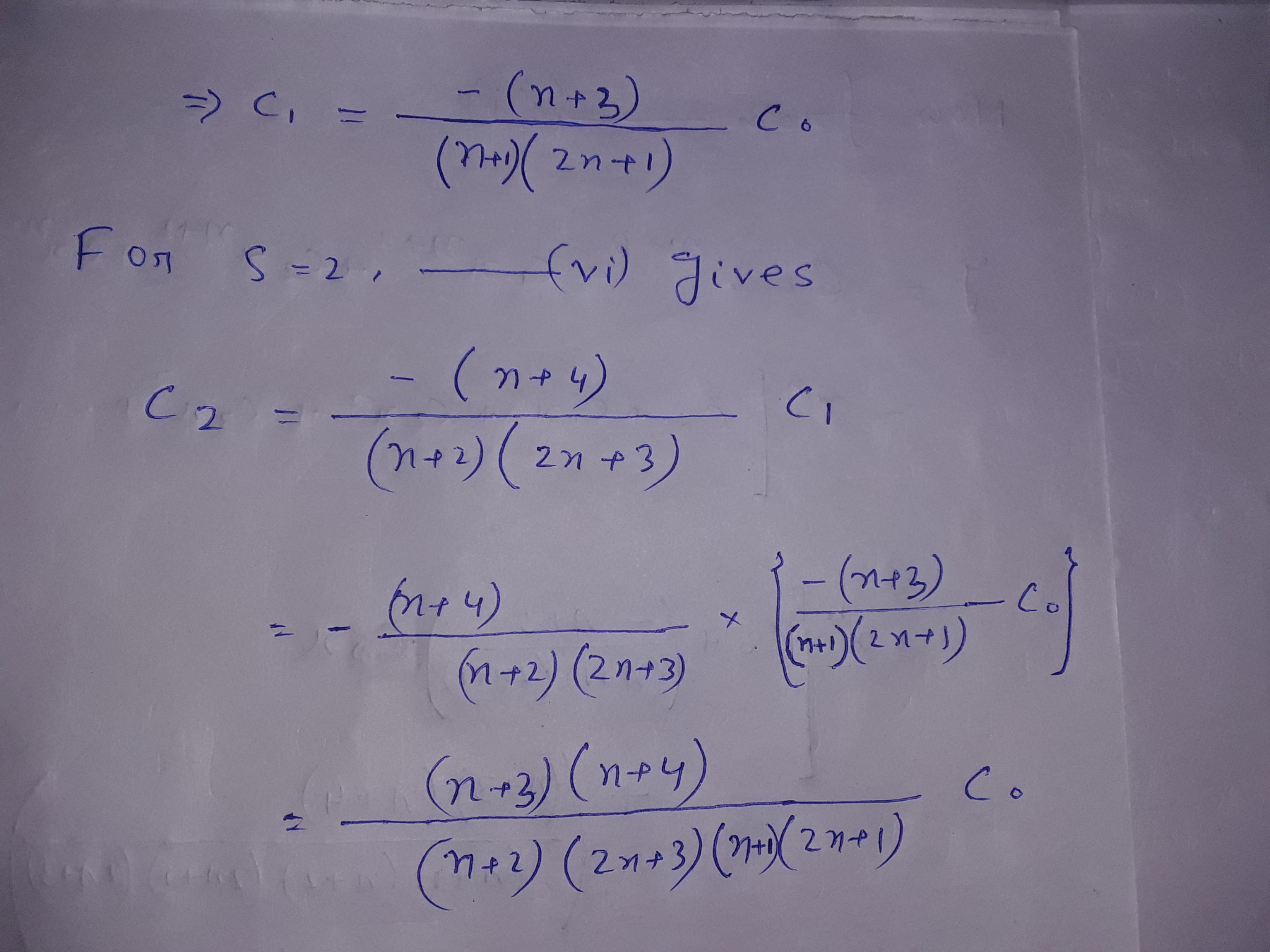Know the answer?
Your Answer:

#### Post as a guest

Your Name:

What's your source?

#### Earn Coin

Coins can be redeemed for fabulous gifts.

Not the answer you're looking for? Ask your own homework help question. Our experts will answer your question WITHIN MINUTES for Free.
Similar Homework Help Questions
• ### In each of Problems 1 through 6: a. Show that the given differential equation has a regular singu...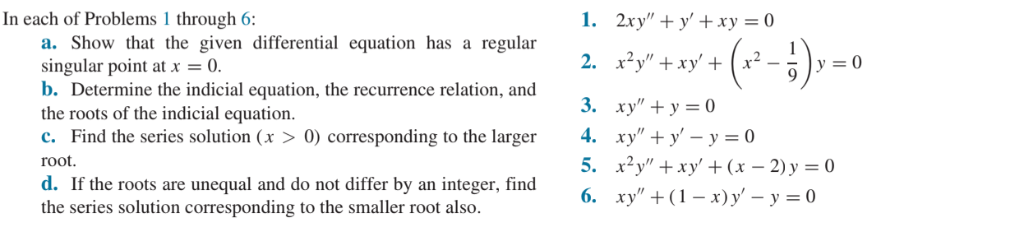Do JUST # 2 please In each of Problems 1 through 6: a. Show that the given differential equation has a regular singular point at x0. b. Determine the indicial equation, the recurrence relation, and the roots of the indicial equation. c. Find the series solution (x >0) corresponding to the larger root. d. If the roots are unequal and do not differ by an integer, find the series solution corresponding to the smaller root also. 2. xy" +xy+ 3....

• ### In each of Problems 1 through 6: a. Show that the given differential equation has a regular singu...Do JUST # 3 Please In each of Problems 1 through 6: a. Show that the given differential equation has a regular singular point at x0. b. Determine the indicial equation, the recurrence relation, and the roots of the indicial equation. c. Find the series solution (x >0) corresponding to the larger root. d. If the roots are unequal and do not differ by an integer, find the series solution corresponding to the smaller root also. 2. xy" +xy+ 3....

• ### Engineering Mathematics IIA Page 3 of 8 3. Consider the second-order ordinary differential equation for y(x)...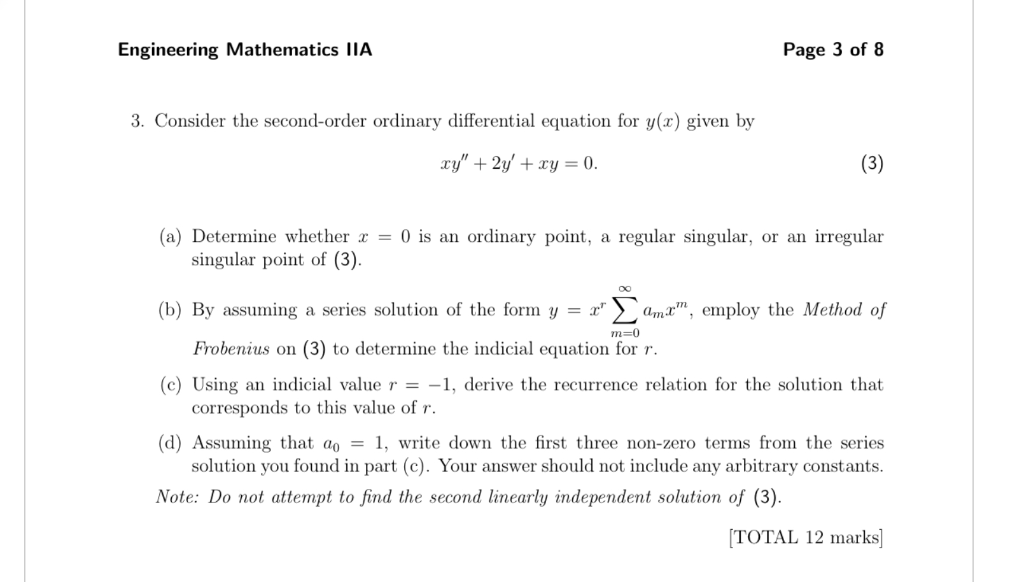Engineering Mathematics IIA Page 3 of 8 3. Consider the second-order ordinary differential equation for y(x) given by (3) xy"2y' +xy = 0. (a) Determine whether = 0 is an ordinary point, regular singular, or an irregular a singular point of (3). (b) By assuming a series solution of the form y = x ama, employ the Method of m-0 Frobenius on (3) to determine the indicial equation for r. (c) Using an indicial value r = -1, derive the...

• ### Question 2 (3 points) For the equation Ir-16"(x-ib"-20' +y-o, the point r-i is 2xy +y -0,...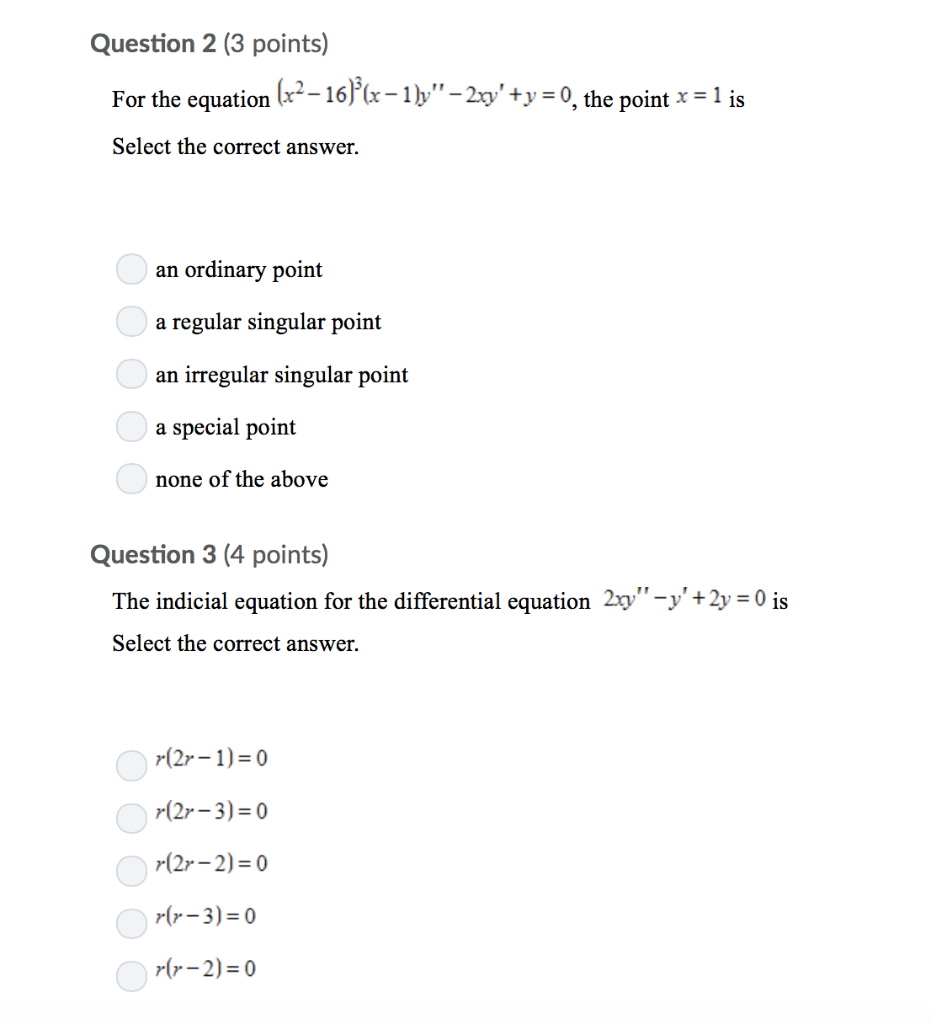Question 2 (3 points) For the equation Ir-16"(x-ib"-20' +y-o, the point r-i is 2xy +y -0, the point x-1 is Select the correct answer. an ordinary point a regular singular point an irregular singular point a special point none of the above Question 3 (4 points) The indicial equation for the differential equation 2xy-'+2y 0 is Select the correct answer. (2r-1)0 (2r-3) 0 (2r-2)-0 rr-3)-0 r(r-2)0

• ### Given that x =0 is a regular singular point of the given differential equation, show that...

Given that x =0 is a regular singular point of the given differential equation, show that the indicial roots of the singularity do not differ by an integer. Use the method of Frobenius to obtain two linearly independent series solutions about x = 0. Form the general solution on (0, ∞) 2xy''-y'+y=0

• ### Consider the following differential equation Note: For each part below you must give your answers in terms of fract...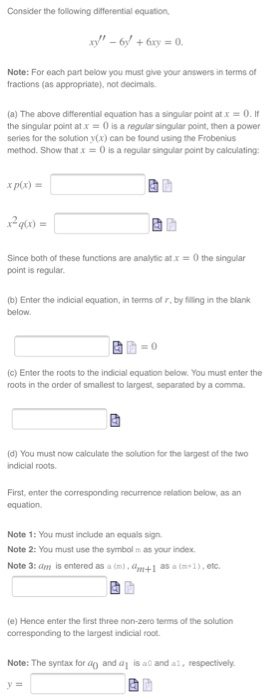Consider the following differential equation Note: For each part below you must give your answers in terms of fractions (as appropriate), not decimals (a) The above differential equation has a snaar point at x 0 . It the singular point at x-0 is a regular singular point, then a power series for the solution y(x) can be lound using the Frobenius method. Show that x = 0 is a regular sigar point by calculating: xp(x) = y(x) = Since both...

• ### Consider the following differential equation Note: For each part below you must give your answers in terms of fractions...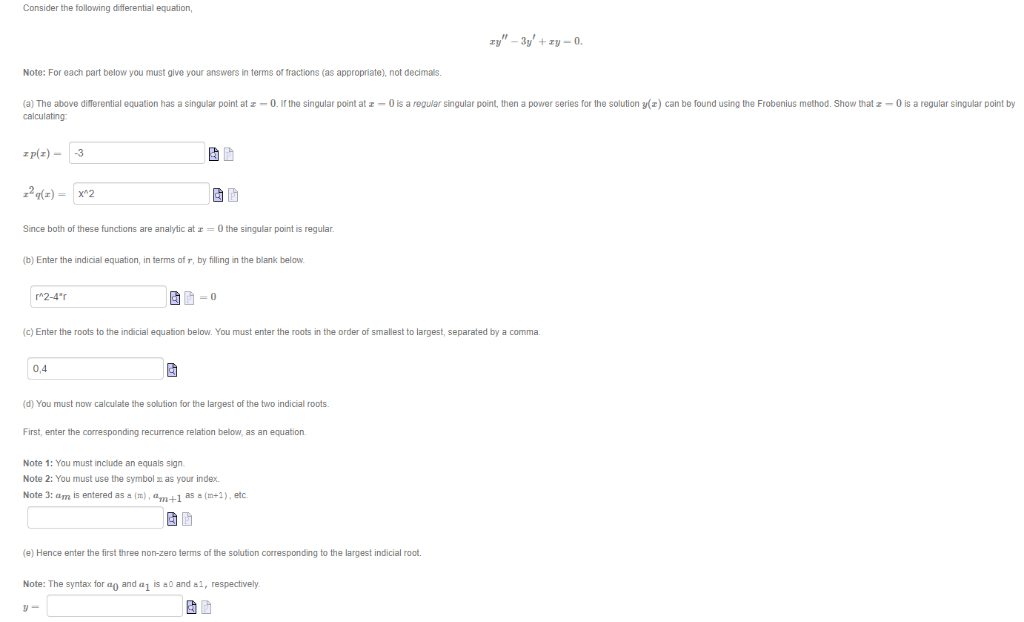Consider the following differential equation Note: For each part below you must give your answers in terms of fractions (as appropriate), not decimas. (a) The above differential equation has a singular point at z-0.I the singular point at z -0 is a regular singular point, then a power series for the solution ()can be found using the Frobenius method. Show that z-O is a regular singular point by calculating plz)-3 Since both of these functions are analytic at r -0...

• ### (20 pts.) The Laguerre differential equation is ry" + (1 - )y' + Ay = 0....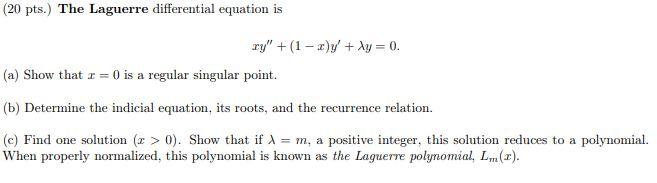(20 pts.) The Laguerre differential equation is ry" + (1 - )y' + Ay = 0. (a) Show that x = 0 is a regular singular point. (b) Determine the indicial equation, its roots, and the recurrence relation. (c) Find one solution (x > 0). Show that if = m, a positive integer, this solution reduces to a polynomial. When properly normalized, this polynomial is known as the Laguerre polynomial, L. (2).

• ### Consider the following differential equation Note: For each part below you must give your answers in terms of fractions...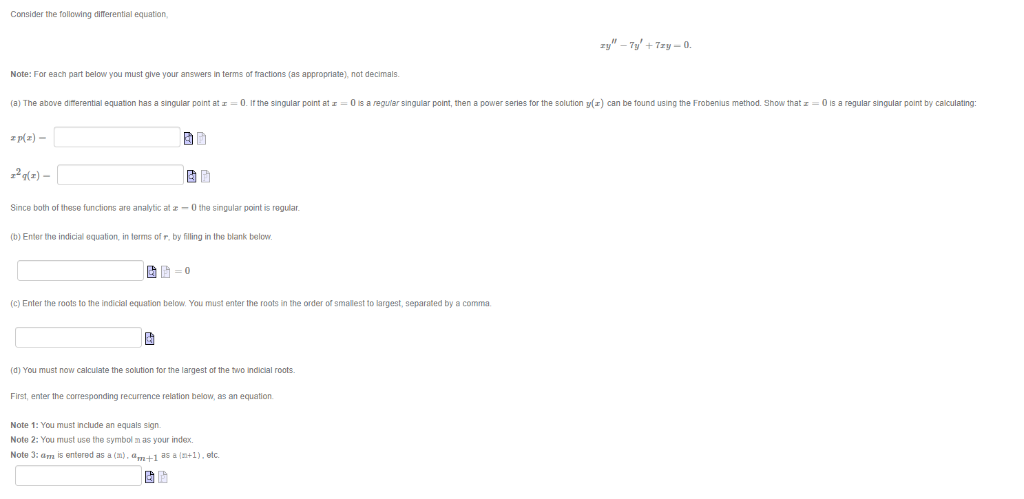Consider the following differential equation Note: For each part below you must give your answers in terms of fractions (as appropriate), not decimals (a) The above difterential equation has a singular point at-0. If the singular point at -0 is a regular singular point, then a power series for the solution y) can be found using the Frobenius method. Show that z-0 is a regular singular point by caliculating p/a)- 2(2) Since both of these functions are analytic at -0...

• ### (1 point) Consider the differential equation 2x(x )y"3 - 1)y -y0 which has a regular singular...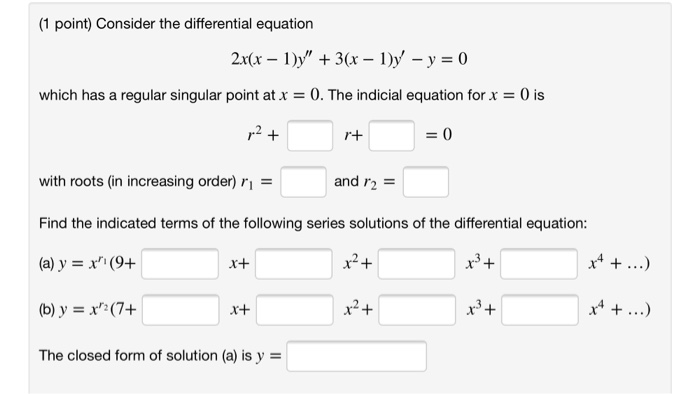(1 point) Consider the differential equation 2x(x )y"3 - 1)y -y0 which has a regular singular point atx 0. The indicial equation for x 0 is 2+ 0 r+ with roots (in increasing order) r and r2 Find the indicated terms of the following series solutions of the differential equation: x4. (a) y =x (9+ x+ (b) y x(7+ The closed form of solution (a) is y (1 point) Consider the differential equation 2x(x )y"3 - 1)y -y0 which has...

Free Homework App

Scan Your Homework
to Get Instant Free Answers
Need Online Homework Help?

Get Answers For Free
Most questions answered within 3 hours.